Learning Library

Money in the Bank: Two-Digit Addition

This little piggy said "wee! wee! wee!" all the way to the bank...because he was loaded! Ask your second grader to do some mental math to figure out how much moolah each pig is carrying.

Click on a worksheet in the set below to see more info or download the PDF.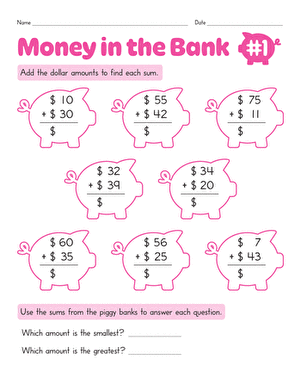Money in the Bank: Two-Digit Addition #1

How much money is in each piggy bank? Give your second grader practice with double-digit addition as he gets more familiar with mental math.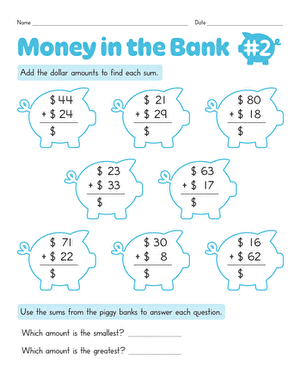Money in the Bank: Two-Digit Addition #2

Use this colorful worksheet to boost your second grader's math skills. She'll add up all the problems to see how much money is in each piggy bank.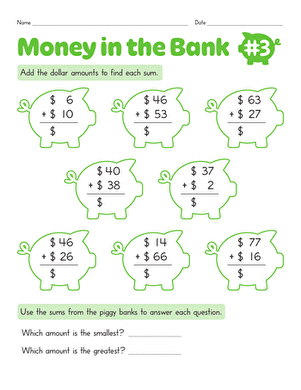Money in the Bank: Two-Digit Addition #3

Don't break the bank! Can your second grader use double-digit addition to determine how much money is in each of these piggy banks?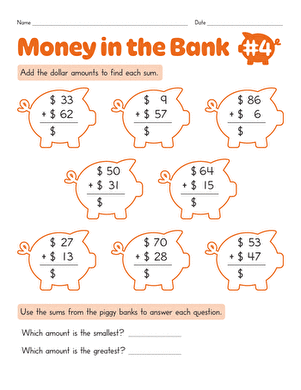Money in the Bank: Two-Digit Addition #4

This cheerful worksheet gives your second grader the practice she needs to master addition!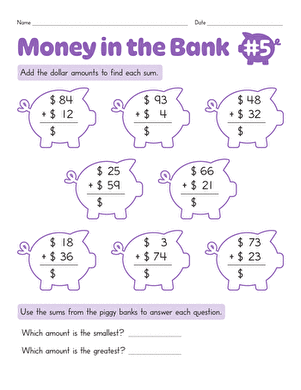Money in the Bank: Two-Digit Addition #5

How much money does each little piggy have? Give your second grader's double-digit addition skills a work out with this fun printable.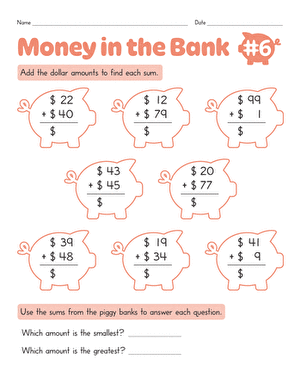Money in the Bank: Two-Digit Addition #6

Can your second grader find out how much money is in each piggy bank? He'll use his addition skills to count up the dollars!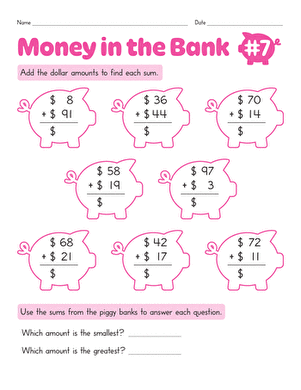Money in the Bank: Two-Digit Addition #7

Which piggy bank has the most money? Your second grader will boost her double-digit addition and mental math ability as she works to find out.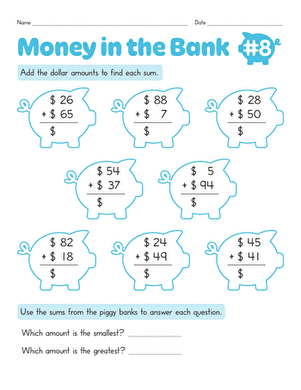Money in the Bank: Two-Digit Addition #8

Which piggy is the richest? Let your second grader use double-digit addition to find out!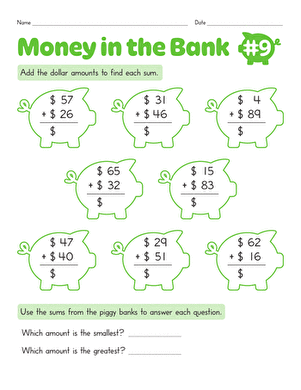Money in the Bank: Two-Digit Addition #9

This charming piggy bank worksheet gives your second grader a chance to exercise his two-digit addition and mental math skills.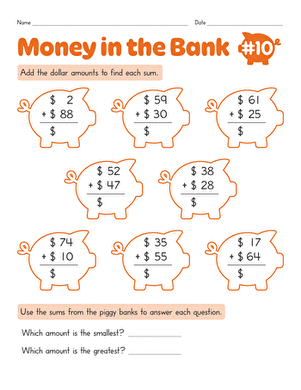Money in the Bank: Two-Digit Addition #10

Your second grader will get a mental math boost as he practices two-digit addition to figure out how much money is in each piggy bank.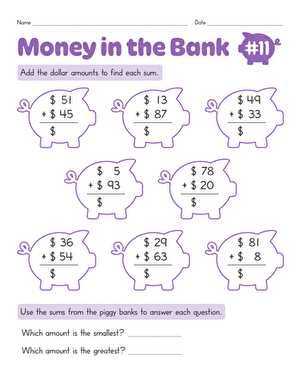Money in the Bank: Two-Digit Addition #11

Which piggy bank has the most money? Help your second grader improve his mental math skills and double-digit addition ability with this colorful printable.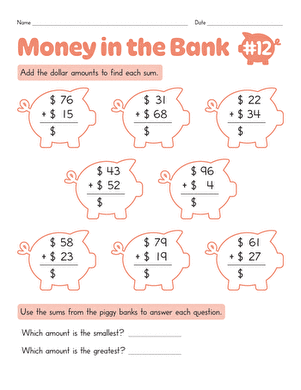Money in the Bank: Two-Digit Addition #12

Watch your second grader boost his mental math skills as he works through these addition problems to find out how much money each piggy bank holds.Money in the Bank: Two-Digit Addition #13

In no time at all, you'll see your second grader boost her double-digit addition skills and practice mental math with this piggy bank worksheet.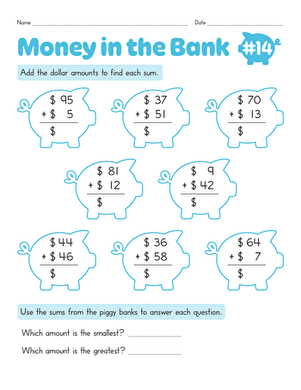Money in the Bank: Two-Digit Addition #14

How many dollars are in each piggy bank? Watch your second grader use his addition skills to count up the dollars, which strengthens his mental math, too.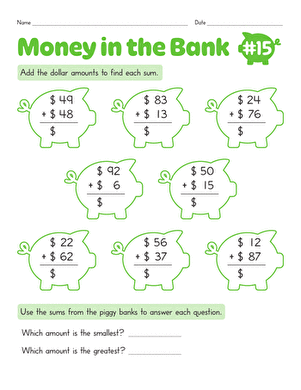Money in the Bank: Two-Digit Addition #15

Let your second grader work through a heap of double-digit addition problems to find the piggy bank that holds the most and least amount of money.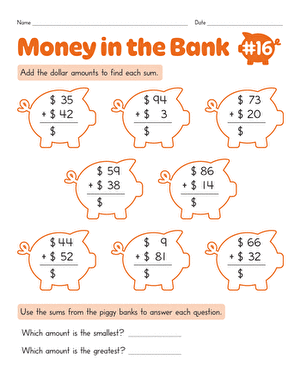Money in the Bank: Two-Digit Addition #16

Kids will solve math problems to find out how much money is in each piggy bank. Exercise mental math skills with this two-digit addition worksheet.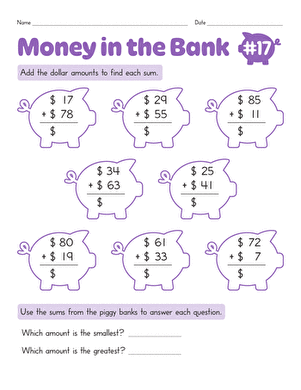Money in the Bank: Two-Digit Addition #17

Find out how much money is in the bank with this two-digit addition piggy bank worksheets. Use mental math to solve addition problems in this math worksheet.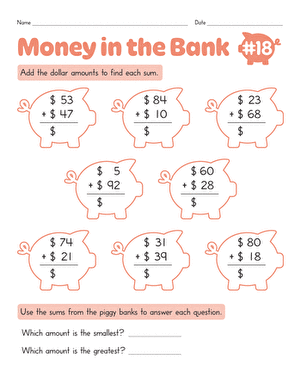Money in the Bank: Two-Digit Addition #18

Use mental math to work through two-digit addition problems in this math worksheet. Kids will solve to find out how much money is in each piggy bank.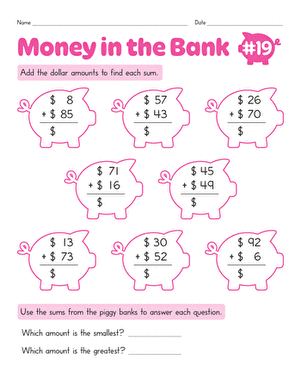Money in the Bank: Two-Digit Addition #19

Use this piggy bank math worksheet to practice two-digit addition. Try this math worksheet with your child.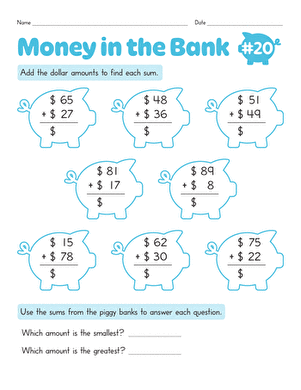Money in the Bank: Two-Digit Addition #20

Work on two-digit addition with this piggy bank math worksheet. Kids will solve problems to find out how much money is in each bank.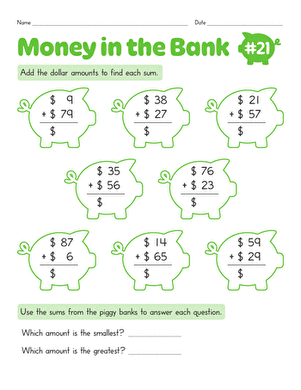Money in the Bank: Two-Digit Addition #21

Solve two-digit addition problems with piggy bank math. Find out how much money is in each piggy bank with this math worksheet.Money in the Bank: Two-Digit Addition #22

Use this colorful two-digit addition worksheet with your child. Solve this addition problems to find out how much money is in each piggy bank.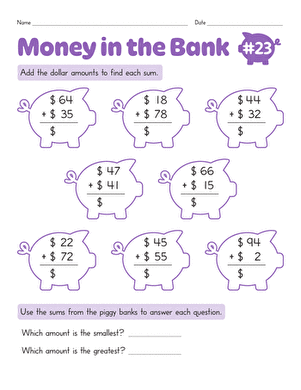Money in the Bank: Two-Digit Addition #23

Try this piggy-bank-themed worksheet to practice addition with your child. Solve addition problems to find out how much money is in each piggy bank.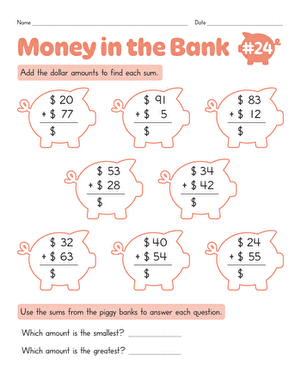Money in the Bank: Two-Digit Addition #24

Kids will do two-digit addition problems to find out how much money is in each piggy bank in this worksheet. Practice math skills with this worksheet.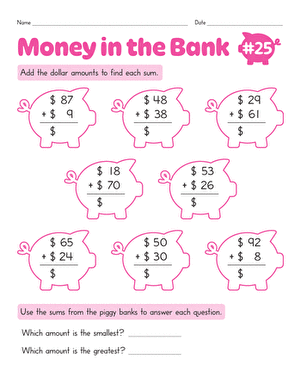Money in the Bank: Two-Digit Addition #25

Solve two-digit addition problems in this piggy-bank-themed worksheet. Use your math skills to figure out how much money is in each piggy bank.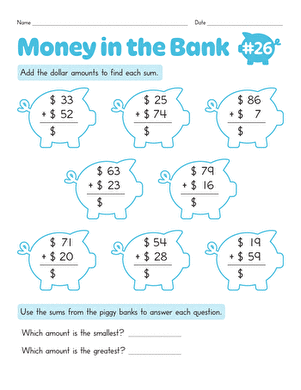Money in the Bank: Two-Digit Addition #26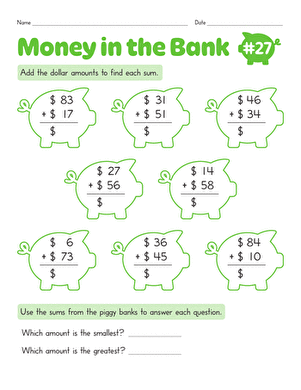Money in the Bank: Two-Digit Addition #27

Kids will solve two-digit addition problems in this piggy-bank math worksheet. Find out how much money is in each piggy bank through addition.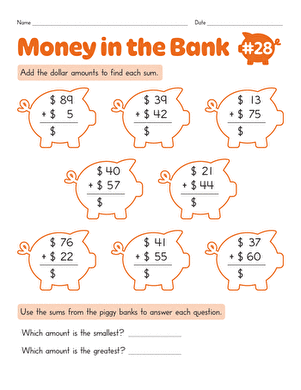Money in the Bank: Two-Digit Addition #28

Use this piggy-bank math worksheet with your young mathematician. Find out how much money is in each piggy with double-digit addition.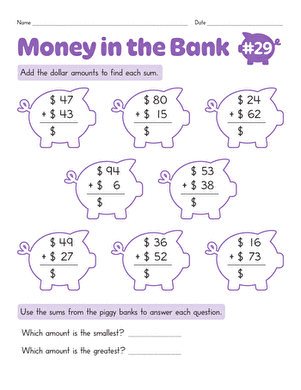Money in the Bank: Two-Digit Addition #29

Try this piggy-bank-themed addition worksheet with your child. Your child will figure out how much money is in each piggy while practicing adding double digits.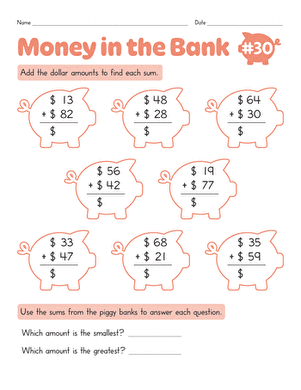Money in the Bank: Two-Digit Addition #30

Help your child put 'money in the bank' with this piggy-bank-themed addition worksheet. Your kid will learn how much money is in each piggy through addition.

Want to download this whole set as a single PDF?

Create new collection

0

New Collection>

0 items

What could we do to improve Education.com?

Please note: Use the Contact Us link at the bottom of our website for account-specific questions or issues.

What would make you love Education.com?